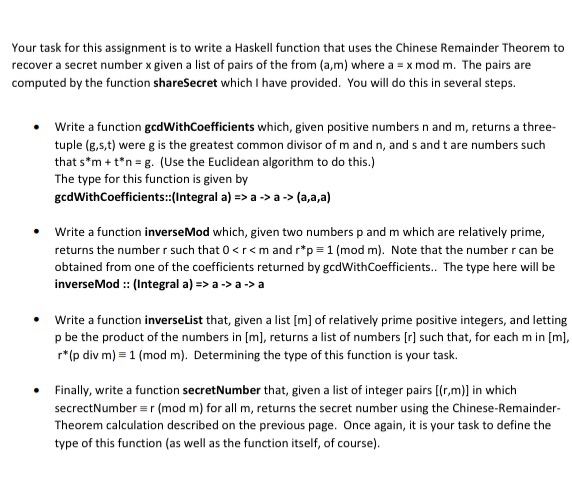# (Solved) : Task Assignment Write Haskell Function Uses Chinese Remainder Theorem Recover Secret Numbe Q42697712 . . .Your task for this assignment is to write a Haskell function that uses the Chinese Remainder Theorem to recover a secret number x given a list of pairs of the from (a,m) where a = x mod m. The pairs are computed by the function shareSecret which I have provided. You will do this in several steps. Write a function gcdWithCoefficients which, given positive numbers n and m, returns a three- tuple (6,s,t) were g is the greatest common divisor of m and n, and s and t are numbers such that s*m + t*n= g. (Use the Euclidean algorithm to do this.) The type for this function is given by gcdWith Coefficients::(Integral a) => a -> -> (a,a,a) Write a function inverse Mod which, given two numbers p and m which are relatively prime, returns the number r such that 0<r<m and r*p= 1 (mod m). Note that the number r can be obtained from one of the coefficients returned by gcdWithCoefficients.. The type here will be inverse Mod :: Integral a) => a ->a-> a Write a function inverselist that, given a list [m] of relatively prime positive integers, and letting p be the product of the numbers in [m], returns a list of numbers [r] such that, for each min [m], rp div m) = 1 (mod m). Determining the type of this function is your task. Finally, write a function secret Number that, given a list of integer pairs [(rm)] in which secrectNumber =r (mod m) for all m, returns the secret number using the Chinese-Remainder- Theorem calculation described on the previous page. Once again, it is your task to define the type of this function (as well as the function itself, of course). Show transcribed image text Your task for this assignment is to write a Haskell function that uses the Chinese Remainder Theorem to recover a secret number x given a list of pairs of the from (a,m) where a = x mod m. The pairs are computed by the function shareSecret which I have provided. You will do this in several steps. Write a function gcdWithCoefficients which, given positive numbers n and m, returns a three- tuple (6,s,t) were g is the greatest common divisor of m and n, and s and t are numbers such that s*m + t*n= g. (Use the Euclidean algorithm to do this.) The type for this function is given by gcdWith Coefficients::(Integral a) => a -> -> (a,a,a) Write a function inverse Mod which, given two numbers p and m which are relatively prime, returns the number r such that 0a-> a Write a function inverselist that, given a list [m] of relatively prime positive integers, and letting p be the product of the numbers in [m], returns a list of numbers [r] such that, for each min [m], rp div m) = 1 (mod m). Determining the type of this function is your task. Finally, write a function secret Number that, given a list of integer pairs [(rm)] in which secrectNumber =r (mod m) for all m, returns the secret number using the Chinese-Remainder- Theorem calculation described on the previous page. Once again, it is your task to define the type of this function (as well as the function itself, of course).

Answer to Your task for this assignment is to write a Haskell function that uses the Chinese Remainder Theorem to recover a secret…

We are the best freelance writing portal. Looking for online writing, editing or proofreading jobs? We have plenty of writing assignments to handle.